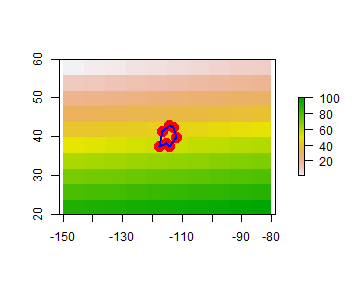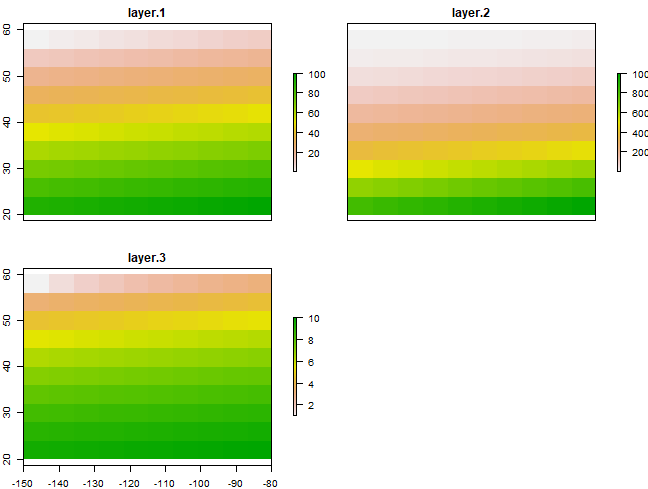# Raster data¶

## Introduction¶

The `sp` package supports raster (gridded) data with with the `SpatialGridDataFrame` and `SpatialPixelsDataFrame` classes. However, we will focus on classes from the `raster` package for raster data. The raster package is built around a number of classes of which the `RasterLayer`, `RasterBrick`, and `RasterStack` classes are the most important. When discussing methods that can operate on all three of these objects, they are referred to as ’Raster*’ objects.

The `raster` package has functions for creating, reading, manipulating, and writing raster data. The package provides, among other things, general raster data manipulation functions that can easily be used to develop more specific functions. For example, there are functions to read a chunk of raster values from a file or to convert cell numbers to coordinates and back. The package also implements raster algebra and many other functions for raster data manipulation.

## RasterLayer¶

A `RasterLayer` object represents single-layer (variable) raster data. A `RasterLayer` object always stores a number of fundamental parameters that describe it. These include the number of columns and rows, the spatial extent, and the Coordinate Reference System. In addition, a `RasterLayer` can store information about the file in which the raster cell values are stored (if there is such a file). A `RasterLayer` can also hold the raster cell values in memory.

Here I create a `RasterLayer` from scratch. But note that in most cases where real data is analyzed, these objects are created from a file.

```library(raster)
r <- raster(ncol=10, nrow=10, xmx=-80, xmn=-150, ymn=20, ymx=60)
r
## class      : RasterLayer
## dimensions : 10, 10, 100  (nrow, ncol, ncell)
## resolution : 7, 4  (x, y)
## extent     : -150, -80, 20, 60  (xmin, xmax, ymin, ymax)
## crs        : +proj=longlat +datum=WGS84 +no_defs
```

Object `r` only has the skeleton of a raster data set. That is, it knows about its location, resolution, etc., but there are no values associated with it. Let’s assign some values. In this case I assign a vector of random numbers with a length that is equal to the number of cells of the RasterLayer.

```values(r) <- runif(ncell(r))
r
## class      : RasterLayer
## dimensions : 10, 10, 100  (nrow, ncol, ncell)
## resolution : 7, 4  (x, y)
## extent     : -150, -80, 20, 60  (xmin, xmax, ymin, ymax)
## crs        : +proj=longlat +datum=WGS84 +no_defs
## source     : memory
## names      : layer
## values     : 0.01307758, 0.9926841  (min, max)
```

You can also assign cell numbers (in this case overwriting the previous values)

```values(r) <- 1:ncell(r)
r
## class      : RasterLayer
## dimensions : 10, 10, 100  (nrow, ncol, ncell)
## resolution : 7, 4  (x, y)
## extent     : -150, -80, 20, 60  (xmin, xmax, ymin, ymax)
## crs        : +proj=longlat +datum=WGS84 +no_defs
## source     : memory
## names      : layer
## values     : 1, 100  (min, max)
```

We can plot this object.

```plot(r)
lon <- c(-116.8, -114.2, -112.9, -111.9, -114.2, -115.4, -117.7)
lat <- c(41.3, 42.9, 42.4, 39.8, 37.6, 38.3, 37.6)
lonlat <- cbind(lon, lat)
pols <- spPolygons(lonlat, crs='+proj=longlat +datum=WGS84')
points(lonlat, col='red', pch=20, cex=3)
```## RasterStack and RasterBrick¶

It is quite common to analyze raster data using single-layer objects. However, in many cases multi-variable raster data sets are used. The `raster` package has two classes for multi-layer data the `RasterStack` and the `RasterBrick`. The principal difference between these two classes is that a `RasterBrick` can only be linked to a single (multi-layer) file. In contrast, a `RasterStack` can be formed from separate files and/or from a few layers (‘bands’) from a single file.

In fact, a `RasterStack` is a collection of `RasterLayer` objects with the same spatial extent and resolution. In essence it is a list of `RasterLayer` objects. A `RasterStack` can easily be formed form a collection of files in different locations and these can be mixed with `RasterLayer` objects that only exist in the RAM memory (not on disk).

A `RasterBrick` is truly a multi-layered object, and processing a `RasterBrick` can be more efficient than processing a `RasterStack` representing the same data. However, it can only refer to a single file. A typical example of such a file would be a multi-band satellite image or the output of a global climate model (with e.g., a time series of temperature values for each day of the year for each raster cell). Methods that operate on `RasterStack` and `RasterBrick` objects typically return a `RasterBrick` object.

Thus, the main difference is that a `RasterStack` is loose collection of `RasterLayer` objects that can refer to different files (but must all have the same extent and resolution), whereas a `RasterBrick` can only point to a single file.

Here is an example how you can make a RasterStack from multiple layers.

```r2 <- r * r
r3  <- sqrt(r)
s <- stack(r, r2, r3)
s
## class      : RasterStack
## dimensions : 10, 10, 100, 3  (nrow, ncol, ncell, nlayers)
## resolution : 7, 4  (x, y)
## extent     : -150, -80, 20, 60  (xmin, xmax, ymin, ymax)
## crs        : +proj=longlat +datum=WGS84 +no_defs
## names      : layer.1, layer.2, layer.3
## min values :       1,       1,       1
## max values :     100,   10000,      10
plot(s)
```And you can make a RasterBrick from a RasterStack.

```b <- brick(s)
b
## class      : RasterBrick
## dimensions : 10, 10, 100, 3  (nrow, ncol, ncell, nlayers)
## resolution : 7, 4  (x, y)
## extent     : -150, -80, 20, 60  (xmin, xmax, ymin, ymax)
## crs        : +proj=longlat +datum=WGS84 +no_defs
## source     : memory
## names      : layer.1, layer.2, layer.3
## min values :       1,       1,       1
## max values :     100,   10000,      10
```